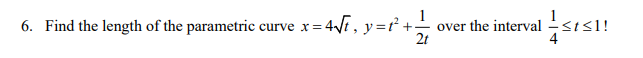### Create an Account

Already have account?

### Forgot Your Password ?

Home / Questions / 6. Find the length of the parametric curve x = 471, y=+ over the interval 2t

# 6. Find the length of the parametric curve x = 471, y=+ over the interval 2t

6. Find the length of the parametric curve x = 471, y=+ over the interval 2t

6. Find the length of the parametric curve x=4sqrt(t), y = t^2+1/2t over the interval 1/4 <= t <= 1Apr 13 2021 View more View Less

#### Answer (Solved)Subscribe To Get Solution